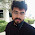# System.out.println(0123)

It was a random meeting with System.out.println(0123)  and after the result, I was completely shocked.I'm shocked because I was expecting the output of this statement is 123 as usual, but what I received is 83.
then I started to find out the reason behind that.

so what I found that if a number is leading with 0 as in my case it was 0123,
in this case, this number is interpreted as Octal, not Decimal.
Octal base(8).
0123 is treated as an octal based value of 123.

so in such a scenario, JVM  print the decimal corresponding value of  0123 which is 83.
example-
System.out.println(012):
``(2 * 8 ^ 0) + (1 * 8 ^ 1) = 10``

128 = 2*80 +1*81 ---> 10

System.out.println(0123)
``(3 * 8 ^ 0) + (2 * 8 ^ 1) + (1 * 8 ^ 2) = 83``

1238 = 3*80 +2*81 +1*82 ---> 83

1.Well explained.

1.2.Very tricky n very common

1.3.in first attempt i was also wrong,
this post helped me to get right answer.

1.4.1.